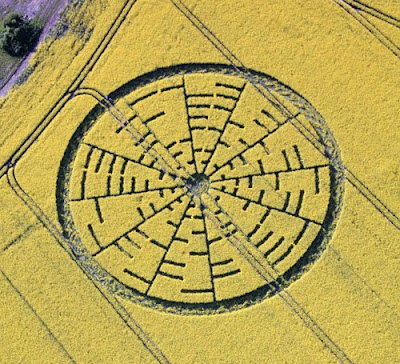## Πέμπτη, 27 Μαΐου 2010

### The 4th Crop Circle in 2010 !!!Explanation : http://logosmythos.net/wilton_windmill_crop_circle_may_2010.html

A new crop picture at Wilton Windmill on May 22, 2010 showed the famous "Euler Identity" from complex mathematics in 8-bit ASCII computer code

A new crop at Wilton Windmill on May 22, 2010 broke new grounds of expression between the crop artists and ourselves, in terms of the sophistication and coding level of their message. Thus it showed a series of 12 x 8 binary digits in standard 8-bit ASCII code (see ASCII), repeated in duplicate within various parts of the message. When translated into modern English, those 96 binary digits give the result:

e ^ (hi)pi) 1 = 0

On our planet, the famous "Euler Identity" from complex mathematics is often written in abbreviated form as (see Euler's_identity):

e ^ (i pi) + 1 = 0

Thus their crop-based message shows two slight differences from what we call the "Euler Identity". First, it does not seem to contain a "plus sign" on the right. Yet if those crop artists were not perfectly familiar with all aspects of our ASCII code, they could easily have written "right parentheses" for "plus" by a single one-bit error. In which case, the crop formula would become:

e ^ (hi)pi + 1 = 0

which is a close fit to our Euler Identity. The second difference is that they seem to write our complex imaginary symbol "i" as "hi".

Many modern scientists and mathematicians regard that Euler Identity to be the "most beautiful mathematical formula ever" (see Leonhard_Euler), or the "greatest equation ever" (see Euler's_identity). It follows as a special case from Euler's more general formula:

e ^ (i x) = cos (x) + i sin (x)

when x = pi. Please look at this great video which explains everything: see www.youtube.com.

"Beautiful!" the author writes there. Yes, it is a simple equation of great mathematical beauty, which is used today in everything from x-ray crystallography to engineering to DNA. And the crop artists showed it to us in a field of oilseed rape in southern England on May 22, 2010. If we cannot now appreciate the great beauty of their high intelligence, and their yearnings to make contact with other advanced scientists or mathematicians on Earth (for whom it was clearly meant), then our local, often-struggling human race here will be the worse for it.

UPDATE:- 25/05/2010
Other mathematical equations related to Euler's Identity

A professional mathematician whom I work with pointed out that "Euler's Identity" is just one of many related equations, some of which are listed below:

e (i 0) - 1 = 0

e (i pi / 2) - i = 0

e (i pi) + 1 = 0 < Euler's Identity (crop picture)

e (3 i pi / 2) + i = 0

e (2 i pi) - 1 = 0

e (5 i pi / 2) - i = 0

(with thanks to M.B.)

CMM Research01100101 e

01011110 ^

00101000 (

01101000 h

01101001 i

00101001 )

01110000 p

01101001 i

00101001 )

00110001 1

00111101 =

00110000 0

Read each 8-bit ASCII character from inside to out, starting along a double tramline which points towards a nearby windmill, using bars = 1 or spaces = 0.

Then proceed clockwise until all twelve 8-bit characters have been read.

In order to read a second duplicate set of binary digits, use bars = 0 or spaces = 1.

Three Relevant Equations

Euler Identity e^(i)pi+1=0

Crop ASCII e^(hi)pi)1=0

Crop ASCII corrected e^(hi)pi+1=0

The ASCII code for right parentheses “)” differs by just one digit from plus “+”, so that is possibly what was meant.

Photographic credit to Lucy Pringle, or decoding credit to “Grail Seeker”.

CMM Research

Euler's Formula

#### 0 σχόλια: